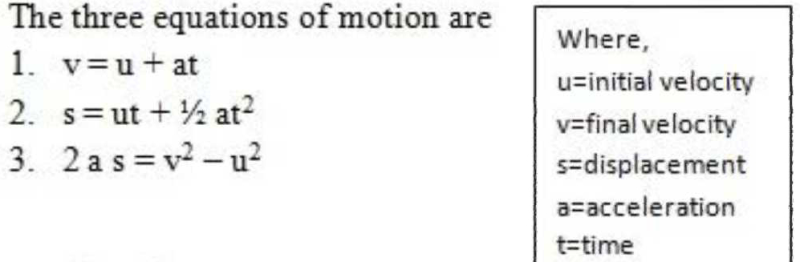Courses

# Equations of motion?

## Class 9 Question

By Aditi Sarda · 3 weeks ago ·Class 9
1.v=u+at
2.s=ut+1/2at^2
3.2as=v^2-u^2Prem Kurakula answered 3 weeks ago
(NOTE:- EQUATIONS OF MOTION ARE ONLY VALID FOR UNIFORM ACCELERATION) .....
1)v=u+at....
2)s=ut+(1/2)at^2....
3)v^2-u^2=2as....
(where v is the final velocity , u is initial velocity , a is acceleration , s is the displacement covered and t is time )

There are 3 equation of motion
1: v=u+at or a=v-u/t.
This equation is known as Velocity-Time
Equation.

2: s=ut+1/2 at(square)
This equation is known as Position-Time Equation

3: v(square)-u(square)=2as
This equation is known as Position-Velocity
Equation

This discussion on Equations of motion? is done on EduRev Study Group by Class 9 Students. The Questions and Answers of Equations of motion? are solved by group of students and teacher of Class 9, which is also the largest student community of Class 9. If the answer is not available please wait for a while and a community member will probably answer this soon. You can study other questions, MCQs, videos and tests for Class 9 on EduRev and even discuss your questions like Equations of motion? over here on EduRev! Apart from being the largest Class 9 community, EduRev has the largest solved Question bank for Class 9.